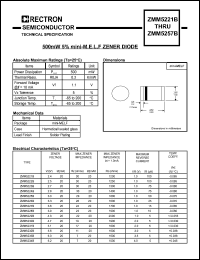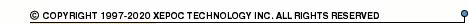More than478 739 registered clientsZMM5221 series datasheets. Manufacturer: RECTR.

 ZMM5221B 5% zener diode. Power dissipation 500 mW. Zener voltage Vz=2.4V at Izt=20mA. Operational temperature range from -65°C to 200°C. Datasheet*) ZMM5222B 5% zener diode. Power dissipation 500 mW. Zener voltage Vz=2.5V at Izt=20mA. Operational temperature range from -65°C to 200°C. Datasheet*) ZMM5223B 5% zener diode. Power dissipation 500 mW. Zener voltage Vz=2.7V at Izt=20mA. Operational temperature range from -65°C to 200°C. Datasheet*) ZMM5224B 5% zener diode. Power dissipation 500 mW. Zener voltage Vz=2.8V at Izt=20mA. Operational temperature range from -65°C to 200°C. Datasheet*) ZMM5225B 5% zener diode. Power dissipation 500 mW. Zener voltage Vz=3.0V at Izt=20mA. Operational temperature range from -65°C to 200°C. Datasheet*) ZMM5226B 5% zener diode. Power dissipation 500 mW. Zener voltage Vz=3.3V at Izt=20mA. Operational temperature range from -65°C to 200°C. Datasheet*) ZMM5227B 5% zener diode. Power dissipation 500 mW. Zener voltage Vz=3.6V at Izt=20mA. Operational temperature range from -65°C to 200°C. Datasheet*) ZMM5228B 5% zener diode. Power dissipation 500 mW. Zener voltage Vz=3.9V at Izt=20mA. Operational temperature range from -65°C to 200°C. Datasheet*) ZMM5229B 5% zener diode. Power dissipation 500 mW. Zener voltage Vz=4.3V at Izt=20mA. Operational temperature range from -65°C to 200°C. Datasheet*) ZMM5230B 5% zener diode. Power dissipation 500 mW. Zener voltage Vz=4.7V at Izt=20mA. Operational temperature range from -65°C to 200°C. Datasheet*) ZMM5231B 5% zener diode. Power dissipation 500 mW. Zener voltage Vz=5.1V at Izt=20mA. Operational temperature range from -65°C to 200°C. Datasheet*) ZMM5232B 5% zener diode. Power dissipation 500 mW. Zener voltage Vz=5.6V at Izt=20mA. Operational temperature range from -65°C to 200°C. Datasheet*) ZMM5233B 5% zener diode. Power dissipation 500 mW. Zener voltage Vz=6.0V at Izt=20mA. Operational temperature range from -65°C to 200°C. Datasheet*) ZMM5234B 5% zener diode. Power dissipation 500 mW. Zener voltage Vz=6.2V at Izt=20mA. Operational temperature range from -65°C to 200°C. Datasheet*) ZMM5235B 5% zener diode. Power dissipation 500 mW. Zener voltage Vz=6.8V at Izt=20mA. Operational temperature range from -65°C to 200°C. Datasheet*) ZMM5236B 5% zener diode. Power dissipation 500 mW. Zener voltage Vz=7.5V at Izt=20mA. Operational temperature range from -65°C to 200°C. Datasheet*) ZMM5237B 5% zener diode. Power dissipation 500 mW. Zener voltage Vz=8.2V at Izt=20mA. Operational temperature range from -65°C to 200°C. Datasheet*) ZMM5238B 5% zener diode. Power dissipation 500 mW. Zener voltage Vz=8.7V at Izt=20mA. Operational temperature range from -65°C to 200°C. Datasheet*) ZMM5239B 5% zener diode. Power dissipation 500 mW. Zener voltage Vz=9.1V at Izt=20mA. Operational temperature range from -65°C to 200°C. Datasheet*) ZMM5240B 5% zener diode. Power dissipation 500 mW. Zener voltage Vz=10V at Izt=20mA. Operational temperature range from -65°C to 200°C. Datasheet*) ZMM5241B 5% zener diode. Power dissipation 500 mW. Zener voltage Vz=11V at Izt=20mA. Operational temperature range from -65°C to 200°C. Datasheet*) ZMM5242B 5% zener diode. Power dissipation 500 mW. Zener voltage Vz=12V at Izt=20mA. Operational temperature range from -65°C to 200°C. Datasheet*) ZMM5243B 5% zener diode. Power dissipation 500 mW. Zener voltage Vz=13V at Izt=9.5mA. Operational temperature range from -65°C to 200°C. Datasheet*) ZMM5244B 5% zener diode. Power dissipation 500 mW. Zener voltage Vz=14V at Izt=9.0mA. Operational temperature range from -65°C to 200°C. Datasheet*) ZMM5245B 5% zener diode. Power dissipation 500 mW. Zener voltage Vz=15V at Izt=8.5mA. Operational temperature range from -65°C to 200°C. Datasheet*) ZMM5246B 5% zener diode. Power dissipation 500 mW. Zener voltage Vz=16V at Izt=7.8mA. Operational temperature range from -65°C to 200°C. Datasheet*) ZMM5247B 5% zener diode. Power dissipation 500 mW. Zener voltage Vz=17V at Izt=7.4mA. Operational temperature range from -65°C to 200°C. Datasheet*) ZMM5248B 5% zener diode. Power dissipation 500 mW. Zener voltage Vz=18V at Izt=7.0mA. Operational temperature range from -65°C to 200°C. Datasheet*) ZMM5249B 5% zener diode. Power dissipation 500 mW. Zener voltage Vz=19V at Izt=6.6mA. Operational temperature range from -65°C to 200°C. Datasheet*) ZMM5250B 5% zener diode. Power dissipation 500 mW. Zener voltage Vz=20V at Izt=6.2mA. Operational temperature range from -65°C to 200°C. Datasheet*) ZMM5251B 5% zener diode. Power dissipation 500 mW. Zener voltage Vz=22V at Izt=5.6mA. Operational temperature range from -65°C to 200°C. Datasheet*) ZMM5252B 5% zener diode. Power dissipation 500 mW. Zener voltage Vz=24V at Izt=5.2mA. Operational temperature range from -65°C to 200°C. Datasheet*) ZMM5253B 5% zener diode. Power dissipation 500 mW. Zener voltage Vz=25V at Izt=5.0mA. Operational temperature range from -65°C to 200°C. Datasheet*) ZMM5254B 5% zener diode. Power dissipation 500 mW. Zener voltage Vz=27V at Izt=4.6mA. Operational temperature range from -65°C to 200°C. Datasheet*) ZMM5255B 5% zener diode. Power dissipation 500 mW. Zener voltage Vz=28V at Izt=4.5mA. Operational temperature range from -65°C to 200°C. Datasheet*) ZMM5256B 5% zener diode. Power dissipation 500 mW. Zener voltage Vz=30V at Izt=4.2mA. Operational temperature range from -65°C to 200°C. Datasheet*) ZMM5257B 5% zener diode. Power dissipation 500 mW. Zener voltage Vz=33V at Izt=3.8mA. Operational temperature range from -65°C to 200°C. Datasheet*) There are other manufacturers for this components. For more information please check ZMM5221 (DIODS) ZMM5221 (EIC) ZMM5221 (JGD)